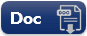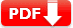# Algebraic Expressions Test 2 – Question Answers

Algebraic Expressions Test 2 – Question Answers: This sample question paper is based on various placement exam in U.S as well as  U.S. Standardized Tests ACCUPLACER, ACT, GED, GMAT, GRE, LSAT, MAT, MCAT, NCLEX, Praxis, SAT, etc

### Algebraic Expressions Practice Test

SET 2

 1. Elijah has a square plot of side m and another triangular plot with base and height each equal to m. What is the total area of both plots? 🔘 A.   \frac{3m^2}{2} 🔘 B.   \frac{2m^3}{3} 🔘 C.   \frac{3m^2}{5} 🔘 D.   \frac{3m}{1} 🔘 E. .   \frac{5m}{3}
Answer: A   \frac{3m^2}{2} (area of square plot + area of triangular plot)
 2. A travel agency charges $8 per km and levies a fixed charge of$ 50 for bus service. Write an algebraic expression for the above situation, if the bus is hired for x km. 🔘 A. 3x + 40 🔘 B. 5x + 27 🔘 C. 2x + 39 🔘 D. 3x + 31 🔘 E. 8x + 50
Answer: E 8x + 50 (algebraic expression (8 × x + 50)
 3. A teacher gets paid $70 per hour. Last week he worked for 9 hours and this week he will work for x hours. Write an algebraic expression for the money paid to him for both the weeks. 🔘 A. 70(x + 9) 🔘 B. x(70 + 9) 🔘 C. 9 (x + 70) 🔘 D. x (70 × 9 ) 🔘 E. 9 (x × 7 ) Show Answers Answer: A 70(x + 9) (Money paid last week 70 × 9 =$630 and money maid this week (70 × x)=$70x, total money paid$630 + 70x = 70(x + 9)
 4. Liam collects 12 leaves and William collects x leaves for science project. After some time Liam loses 3 leaves and William collects 2x leaves. Which one of the following algebraic expression of the total number of leaves collected by both of them. 🔘 A. 12 + 2x 🔘 B. 12-9 + 3x 🔘 C. 7 + 3x 🔘 D. 3 + 3x 🔘 E. 9 + 3x
Answer: E 9 + 3x (Liam collected 12-3=9 leaves and  William x + 2x =3x  so total is 9 + 3x
 5.Simplify combining like terms z2 + 13z2 – 5z + 7z3 – 15z 🔘 A. 7z3 + 12z2 -20z 🔘 B. 5z2 + 3z2 -11z 🔘 C. 5z2 + 6z3 -10z 🔘 D. 9z3 + 8z2 -6z 🔘 E. 3z2 + 7z2 -13z
Answer: A. 7z3 + 12z2 -20z
 6.  Simplify combining like terms 5x2y – 5x2 + 3yx2 – 3y2 + x2 – y2 + 8xy2 – 3y2 🔘 A. 8x2y + 8xy2 – 4x2 – 7y2 🔘 B. 4x2y + 4xy2 – 2x2 – 3y2 🔘 C. 2x2y + 2xy2 🔘 D. 3x2 – 5y2 🔘 E. 5xy2 – 3x2 – 2y2
Answer: A 8x2y + 8xy2 – 4x2 – 7y2
 7.  Add ab – 4a, 4b – ab, 4a – 4b 🔘 A. 1 🔘 B. 2 🔘 C. 3 🔘 D. 4 🔘 E. 0
 Document Type Download Link Free Editable Doc FileFree Printable PDF File